# On the sum of three arbitrary Fibonacci and Lucas numbers

Nurettin Irmak, Zafer Şiar and Refik Keskin
Notes on Number Theory and Discrete Mathematics
Print ISSN 1310–5132, Online ISSN 2367–8275
Volume 25, 2019, Number 4, Pages 96–101
DOI: 10.7546/nntdm.2019.25.4.96-101
Download full paper: PDF, 135 Kb

## Details

### Authors and affiliations

Nurettin IrmakDepartment of Mathematics, Ömer Halisdemir University Niğde, Turkey

Zafer ŞiarDepartment of Mathematics, Bingöl University Bingöl, Turkey

Refik KeskinDepartment of Mathematics, Sakarya University Sakarya, Turkey

### Abstract

In this paper, we solve the equations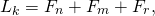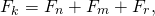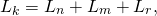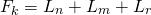for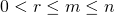and a natural number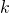. It is shown that only the equation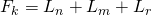has a finite number of solutions. The others have infinitely many solutions.

### Keywords

• Fibonacci number
• Lucas number
• Rank
• Recurrences

• 11B39
• 11D61
• 11B37

### References

1. Bugeaud, Y., Mignotte, M. & Siksek, S. (2006). Classical and modular approaches to exponential Diophantine equations I. Fibonacci and Lucas perfect powers, Annals of Mathematics, 163 (3), 969–1018.
2. Carlitz, L. (1964). A note on Fibonacci numbers,The Fibonacci Quarterly, 2 (1), 15–28.
3. Farrokhi, D. G. M. (2007). Some remarks on the equation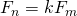in Fibonacci numbers,Journal of Integer Sequences, 10, Article 07.5.7.
4. Keskin, R. & Demirtürk Bitim, B. (2011). Fibonacci and Lucas congruences and their applications, Acta Mathematica Sinica (English Series), 27(4), 725–736.
5. Koshy, T. (2001). Fibonacci and Lucas Numbers with Applications, John Wiley and Sons, Proc., New York-Toronto.
6. Luca, F., & Szalay, L. (2009). Lucas Diophantine Triples,Integers, 9, 441–457.

## Cite this paper

APA

Irmak, N., Şiar, Z. & Keskin, R. (2019). On the sum of three arbitrary Fibonacci and Lucas numbers. Notes on Number Theory and Discrete Mathematics, 25(4), 96-101, doi: 10.7546/nntdm.2019.25.4.96-101.

Chicago

Irmak, Nurettin, Zafer Şiar and Refik Keskin. “On the Sum of Three Arbitrary Fibonacci and Lucas Numbers.” Notes on Number Theory and Discrete Mathematics 25, no. 4 (2019): 96-101, doi: 10.7546/nntdm.2019.25.4.96-101.

MLA

Irmak, Nurettin, Zafer Şiar and Refik Keskin. “On the Sum of Three Arbitrary Fibonacci and Lucas Numbers.” Notes on Number Theory and Discrete Mathematics 25.4 (2019): 96-101. Print, doi: 10.7546/nntdm.2019.25.4.96-101.

Comments are closed.